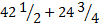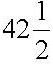# User Forum

Subject :IMO    Class : Class 4

Class : Class 1

(C):

1 kg = 1000g
Cost of 1 kg i.e 1000g oranges = Rs 120
Cost of 250g oranges = (120 / 1000) x 250 = Rs 30
Cost of 1kg  i.e 1000g apples = Rs 150
Cost of 500g apples = (150 / 1000) x 500 = Rs 75
Cost of 250g  oranges and 500g apples altogether = 30 + 75 = Rs 105

Subject :IMO    Class : Class 7

## Ans 1:

Class : Class 8

Subject :IMO    Class : Class 2

## Ans 1:

Class : Class 3

Subject :IMO    Class : Class 9

Subject :IMO    Class : Class 5

## Ans 1:

Class : Class 5

Subject :IMO    Class : Class 7

Class : Class 1

(C)

She will be facing North West after turning 45° anticlockwise. After turning 135° clockwise she will be facing East.
Finally after turning 90° clockwise she will be facing South.

Subject :IMO    Class : Class 7

Class : Class 1

(D): Quantity of juice Shivam purchased in two days == 85/2 + 99/4
= ( 170 + 99) / 4
= 269/4
= 67 1/4 litres
67 ¼ litres is approximately equal to 67 litres.

Subject :IMO    Class : Class 7

Class : Class 1

(D)

Number of fruits liked by A = 9, B =8, G = 8, M =10 and O = 7.
Answer-(D) B and G are liked by an equal number of students.

Subject :IMO    Class : Class 7

Class : Class 1

Step -4 Multiply both side by 4 is incorrect. It should be divide both sides by 4.

Subject :IMO    Class : Class 7

Class : Class 1

(A)

Cost of 12kg of coffee she sold = 12 x 56 = Rs. 672
Cost of 18kg of coffee she sold = 18 x 46 = Rs. 828
Average selling price=  Rs 50